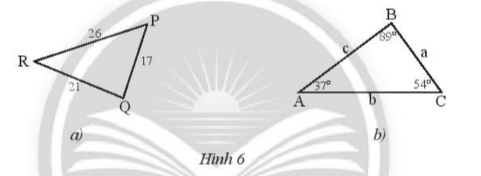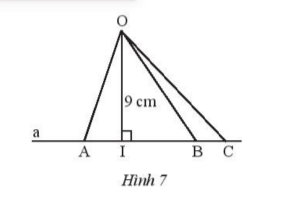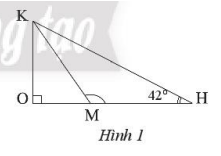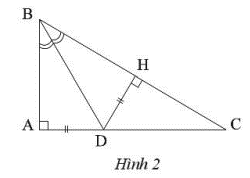## Solving SBT Lesson 4: Perpendicular and oblique lines (C8 SBT Math 7 Horizon) – Math Book

Solving SBT Lesson 4: Perpendicular and oblique lines (C8 SBT Math 7 Horizon)
===========

### Solve problem 1 page 52 SBT Math 7 Creative horizon episode 2 – CTST

a) Arrange in order from smallest to largest the measures of the angles of the triangle PQR figure 6a.

b) Arrange in order from smallest to largest the lengths of the sides of triangle ABC in figure 6b.Detailed instructions for solving Lesson 1

Solution method

– The relationship between sides and angles in a triangle to arrange the measures of angles and sides of a triangle

Detailed explanation

a) Considering triangle PRQ we have: PR > RQ > QP infer \(\widehat Q > \widehat P > \widehat R\)

b) Considering triangle ABC, we have: \(\widehat B > \widehat C > \widehat {{A^{}}}\)infer b > c > a

–>

— *****

### Solve problem 2 page 52 SBT Math 7 Creative horizon episode 2 – CTST

a) Let a triangle DEF whose angle F is an obtuse angle. In your opinion, which side is the longest of the three sides of triangle DEF.

b) Let ABC be a right triangle at A. In your opinion, which side is the longest of the three sides of triangle ABC?

Detailed instructions for solving Lesson 2

Solution method

Compare angles opposite sides to find the longest side.

Detailed explanation

a) In triangle DEF with obtuse F is the largest angle, the opposite side DE is the longest side.

b) In triangle ABC, right angle A is the largest angle, so the hypotenuse BC is the longest side.

–>

— *****

### Solution 3 page 52 SBT Math 7 Creative horizon episode 2 – CTST

In figure 7,

a) Find the shortest path among the lines OA, OI, OB, OC.

b) Find the distance from O to the line a.Detailed instructions for solving Lesson 4

Solution method

– Use the perpendicular and oblique line relationship to find the shortest path.

– The distance from O to the straight line a is the perpendicular from O to the line a.

Detailed explanation

a) The lines OA, OI, OB, OC only have OI which is perpendicular to line a, so the line OI is the shortest.

b) The distance from O to a is equal to OI = 9 cm.

–>

— *****

### Solution 4 page 52 SBT Math 7 Creative horizon episode 2 – CTST

Let the triangle MNP have \(\widehat M = {120^o},\widehat N = {30^o}\)

a) Find the largest side of the triangle MNP.

b) What is the triangle MNP? Why?

Detailed instructions for solving Lesson 4

Solution method

– Find the largest angle in the triangle to deduce the largest side of the triangle MNP.

– Prove \(\widehat P = \widehat N\) to deduce that triangle MNP is an isosceles triangle at M.

Detailed explanation

a) In triangle MNP, angle M is the largest angle, so the side opposite NP is the largest side of triangle MNP.

b) We have: \(\widehat M + \widehat N + \widehat P = {180^o}\) so \(\widehat P = {180^o} – \widehat M – \widehat N = {180 ^o} – {120^o} – {30^o} = {30^o}\) so \(\widehat P = \widehat N\) infer that the triangle MNP is isosceles at M.

–>

— *****

### Solve problem 5 pages 53 SBT Math 7 Creative horizon episode 2 – CTST

Let OHK be a right triangle at O ​​with \(\widehat H = {120^o}\).

a) Compare the sides of the triangle.

b) Take any point M on segment OH. Compare the lengths of KM and KH.

Detailed instructions for solving Lesson 5

Solution method

– Compare the angles opposite the sides and then infer the sides in the triangle OHK.

– Compare the angles opposite sides KM and KH and then compare the lengths KM and KH

Detailed explanationa) We have in triangle OHK right-angled at O:

\(\widehat O + \widehat K + \widehat H = {180^o}\) deduce \(\widehat K = {180^o} – \widehat O – \widehat H = {180^o} – { 120^o} – {42^o} = {48^o}\)

Hence: \(\widehat O > \widehat K > \widehat H\)

Infer: KH > OH > OK.

b) Considering triangle KMH, we have angle KMH as obtuse angle, infer that side KH is the longest side. So Customer > KM

–>

— *****

### Solution 6 page 53 SBT Math 7 Creative horizon episode 2 – CTST

Let ABC be a right triangle at A. The bisector of \(\widehat B\) cuts AC at D. Compare the lengths AD and DC.

Detailed instructions for solving Lesson 6

Solution method

– Use the relationship between the perpendicular and the oblique line to compare the lengths of AD and DC.

Detailed explanationDraw DH perpendicular to BC

We have: DH = DA

In right triangle DHC we have DC as hypotenuse, infer DC > DH.